Next: 5.1.2 Elastic Model with Up: 5.1 Mechanical Models Previous: 5.1 Mechanical Models

## 5.1.1 Elastic Model

In this model the materials are treated as elastic solids parameterized by their Young Modulus E and Poisson ratio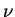. The stress tensor is calculated uniquely from the strain tensor which is solved from the Navier Stokes equations [Zie91] together with the displacement boundary conditions.

In theory of linear elasticity with small displacements the strain tensor can be defined as=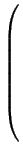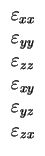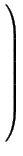=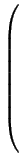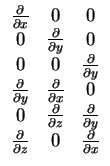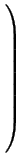.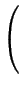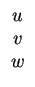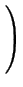= L .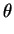(5.1)

with the displacement field:(x, y, z) =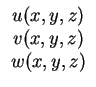(5.2)

Assuming a linear material law, the stress tensor can now be calculated using the equation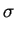= D . (-) +(5.3)

whereare prestrains andare prestresses due to change of temperature, crystal growth or for, e.g., volumetric expansions as in case of oxidation. Assuming an isotropic material (5.3) can be written as: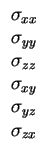=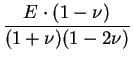.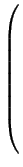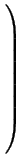(5.4)

Introducing the distributed body forces

 f (x, y, z) =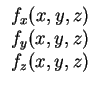(5.5)

the system of differential equations to be solved is finally found to: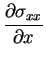+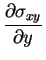+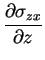=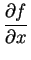(5.6)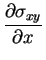+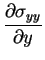+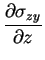=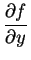(5.7)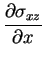+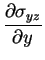+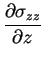=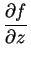(5.8)Next: 5.1.2 Elastic Model with Up: 5.1 Mechanical Models Previous: 5.1 Mechanical Models